# KVPY-SX 2017 Maths Paper with Solutions

Check the KVPY-SX 2017 Maths question paper and solutions on this page. Solving the previous year question paper is one of the best ways to prepare for the KVPY exam. These solutions will help students to understand the marking scheme and exam pattern. Students can easily download the solutions in PDF format also. Solving these solutions will help students to improve their problem-solving skills.

### KVPY-SX 2017 - Maths

Question 1: Let BC be a fixed-line segment in the plane. The locus of a point A such that the triangle ABC is isosceles, is (with finitely many possible exceptional points)

1. a. A line
2. b. A circle
3. c. The union of a circle and a line
4. d. The union of two circles and a line

Solution:

Let the coordinates of fixed base BC be B (0, 0), C (a, 0) and the variable point A (h, k).

Since △ABC is isosceles, three cases arises:

Case-I: AB = AC

√h2 + k2 = √(h - a)2 + k2

h2 + k2 = h2 + a2 – 2ah + k2

h = a / 2

x = a / 2

Locus of A is straight line,

Case-II: AB = BC

√h2 + k2 = a

h2 + k2 = a2

x2 + y2 = a2

Circle

Case-III: AB = BC

√(h - a)2 + k2 = a

(h – a)2 + k2 = a2

(x – a)2 + k2 = a2

Circle

So, the locus of A is union of two circles and a line.

Question 2: The number of solution pairs (x, y) of the simultaneous equations log1/3(x + y) + log3(x – y) = 2 and 2y2 = 512x+1 is

1. a. 0
2. b. 1
3. c. 2
4. d. 3

Solution:

Given, log1/3(x + y) + log3(x – y) = 2

log3^(-1) (x + y) + log3 (x – y) = 2

–log3 (x + y) + log3 (x – y) = 2 {logb^n a = (1 / n) logba}

log3 [(x - y) / (x + y)] = 2 {log m – log n = log m / n}

Taking antilog

(x - y) / (x + y) = 9

x – y = 9x + 9y

8x + 10y = 0

4x + 5y = 0 …(i)

Also, 2y^2 = 512x+1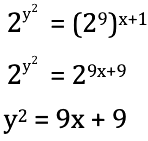----- (ii)

From eq.(i) & (ii)

y2 = 9 (-5y / 4) + 9

4y2 + 45y – 36 = 0

4y2 + 48y – 3y – 36 = 0

(4y – 3) (y + 12) = 0

y = 3 / 4, –12

Using (i)

x = – 15 / 16 , 15

We have (–15 / 16, 3 / 4) and (15, –12)

Since, (–15 / 16, 3 / 4) do not satisfy the original equation, rejected.

So the only answer is (15, –12).

Question 3: The value of the limit limx→∞ (√(4x2 - x) + 2x) is

1. a. –∞
2. b. − 1 / 4
3. c. 0
4. d. 1 / 4

Solution:

limx→∞ (√(4x2 - x) + 2x)

Put x = –y; x → –∞, y → +∞

Therefore limy→∞ (√(4y2 + y) – 2y)

Rationalizing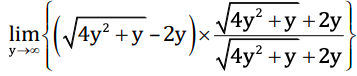limy→∞ (y / √(4y2 + y) + 2y)

Dividing numerator and denominator by y

limy→∞ 1 / [√(4 + [1 / y])] + 2

= 1 / [√(4 + 0) + 2] {1 / ∞ → 0}

= 1 / 4

Question 4: Let R be a relation on the set of all-natural numbers given by a R b ⇔ a divides b2. Which of the following properties does R satisfy?

I. Reflexivity

II. Symmetry

III. Transitivity

1. a. I only
2. b. III only
3. c. I and III only
4. d. I and II only

Solution:

R: N → N, aRb a R a ⇒ a divides b2

Reflexivity: a R a ⇒ a divides a2

True, for every natural number.

‘R’ is reflexive.

Symmetry: a R b ⇒ a divides b2

⇏ b divides a2

For example: 1 R 2 i.e. 1 divides 22, but 2 R 1 i.e. 2 does not divides 12.

‘R’ is not symmetric.

Transitivity:

Again by taking example,

8 R 4 i.e. 8 divides 42 = 16

4 R 2 i.e. 4 divides 22 = 4

But 8 R 2 i.e. 8 do not divide 22 = 4

R is not transitive.

Question 5: The fractional part of a real number x is x – [x], where [x] is the greatest integer less than or equal to x. Let F1 and F2 be the fractional parts of (44 – √2017)2017 and (44 + √2017)2017 respectively. Then F1 + F2 lies between the numbers

1. a. 0 and 0.45
2. b. 0.45 and 0.9
3. c. 0.9 and 1.35
4. d. 1.35 and 1.8

Solution:

Let x = (44 – √2017)2017

x = – (√2017 – 44)2017 ≅ – (0.911)2017

–x = (√2017 – 44)2017 {Pure fractional number}

{–x} = {(√2017 – 44)2017}

1 – {x} = (√2017 – 44)2017

1 – F1 = (√2017 – 44)2017 …(1)

Again, let y = (√2017 + 44)2017 = I + F2 …(2)

Where [y] = I & {y} = F2

Now subtracting eq.(2) – eq.(1)

I + F2 – 1 + F1 = (√2017 + 44)2017 – (√2017 – 44)2017

= 2{2017C1(√2017) 2016(44)1 + ……}

= 2 (Integer)

F1 + F2 = 2I1 – I + 1 ∈ Integer

= 1 (only possible value)

0.9 < F1 + F2 < 1.35

Question 6: The number of real solutions of the equation 2sin 3x + sin 7x – 3 = 0 which lie in the interval [–2𝛑, 2𝛑] is

1. a. 1
2. b. 2
3. c. 3
4. d. 4

Solution:

2 sin3x + sin 7x – 3 = 0 ………(1)

Since maximum value of sin θ is 1, therefore only possible solution of (1) is,

sin3x = 1 and sin7x = 1

3x = (4n + 1) 𝛑 / 2, n ∈ I and 7x = (4m + 1) 𝛑 / 2, m ∈ I

x = (4n + 1) 𝛑 / 6, n ∈ I

x = (4m + 1) 𝛑 / 14, m ∈ I

x = …., – 7𝛑 / 6, – 𝛑 / 2, 𝛑 / 6, 5𝛑 / 6, 3𝛑 / 2 …..

x = …, – 3𝛑 / 14, – 𝛑 / 2, 𝛑 / 14, 5𝛑 / 14, 13𝛑 / 14, 3𝛑 / 2 …

Therefore, two solutions, x = – 𝛑 / 2 and 3𝛑 / 2.

Question 7: Suppose p, q, r are real numbers such that q = p (4 – p), r = q (4 – q), p = r (4 – r). The maximum possible value of p + q + r is

1. a. 0
2. b. 3
3. c. 9
4. d. 27

Solution:

Given, q = p (4 – p) ⇒ q / p = 4 – p ..……(i)

r = q (4 – q) ⇒ r / q = 4 – q ……. (ii)

p = r (4 – r) ⇒ p / r = 4 – r ……..(iii)

Multiplying (i),(ii) and (iii)

(q / p) . (r / q) . (p / r) = (4 – p) (4 – q) (4 – r) = 1 ……..(iv)

Let x = 4 – p = 4 – 4r + r2 = (r – 2)2 ≥ 0

y = 4 – q = 4 – 4p + p2 = (r – 2)2 ≥ 0

z = 4 – r = 4 – 4q + q2 = (q – 2)2 ≥ 0

Now,

Using A.M. ≥ G.M.

(x + y + z) / 3 ≥ (x . y . z)

[4 – p + 4 – q + 4 – r] / 3 ≥ {(4 – p) (4 – q) (4 – r)}

4 – p + 4 – q + 4 – r ≥ 3 {Using eq.(iv)}

p + q + r ≤ 9 (Equality holds for p = q = r = 3)

Question 8: The parabola y2 = 4x + 1 divides the disc x2 + y2 ≤ 1 into two regions with areas A1 and A2. Then |A1 – A2| equals

1. a. 1 / 3
2. b. 2 / 3
3. c. 𝛑 / 4
4. d. 𝛑 / 3

Solution: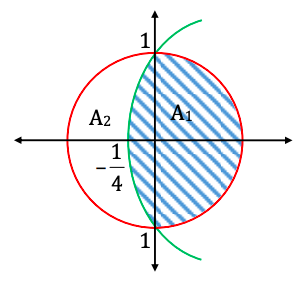C1: y2 = 4x + 1; C2: x2 + y2 = 1

A1 = 2 ∫-1/40 √4x + 1 dx + 2 ∫01 √1 - x2 dx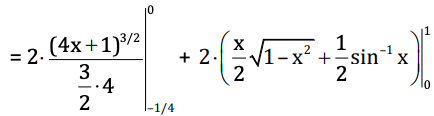= 2 [(1 / 6) – 0] + 2 [0 + (1 / 2) * (𝛑 / 2) – 0]

= 1 / 3 + π / 2

A2 = π(1)2 – A1

Now,

|A1 - A2| = |2A1 - π| = | 2 * [(1 / 3) + (π / 2)] - π | = 2 / 3

Question 9: A shooter can hit a given target with probability 1 / 4. She keeps firing a bullet at the target until she hits it successfully three times and then she stops firing. The probability that she fires exactly six bullets lies in the interval

1. a. (0.5272, 0.5274)
2. b. (0.2636, 0.2638)
3. c. (0.1317, 0.1319)
4. d. (0.0658, 0.0660)

Solution:

Since she hits the target successfully three times in exactly six attempts.

So 3rd hit must be the sixth time and in the first five attempts, there will be two hits. Now, Required Probability =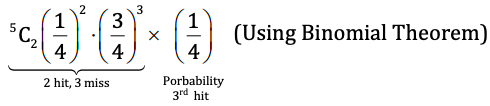= 10 × [27 / 46] = 270 / 4096 = 0.06591

Question 10: Consider the following events:

E1: Six fair dice are rolled and at least one dice shows six.

E2: Twelve fair dice are rolled and at least two dice show six.

Let p1 be the probability of E1 and p2 be the probability of E2.

Which of the following is true?

1. a. p1 > p2
2. b. p1 = p2 = 0.6651
3. c. p1 < p2
4. d. p1 = p2 = 0.3349

Solution:

E1: Six fair dice are rolled and at least one dice shows six.

E2: Twelve fair dice are rolled and at least two dice show six.

P(E1) = p1 = 1 – {no dice shows six}

= 1 – (5 / 6)6 (Using Binomial Theorem)

= 0.6651

P(E2) = p2 = 1 – {no dice shows six + one dice shows six}

= 1 – {(5 / 6)12 + 12C1 (1 / 6) (5 / 6)11} (Using Binomial Theorem)

= 0.61866

p1 > p2

Question 11: For how many different values of a does the following system have at least two distinct solutions? ax + y = 0

x + (a + 10) y = 0

1. a. 0
2. b. 1
3. c. 2
4. d. Infinitely many

Solution:

ax + y = 0; x + (a + 10) y = 0

The given system is homogeneous system of linear equations in two variables Therefore, it will either have unique solution i.e. x = y = 0 or infinite number of solutions (when lines are coincident).

Atleast two distinct solutions → Infinite solutions

a / 1 = 1 / [a + 10] (Co-incident lines)

a2 + 10a – 1 = 0 (Discriminant > 0)

Therefore, two values of ‘a’ are possible.

Question 12: Let R be the set of real numbers and f : R →R be defined by f (x) = {x} / [1 + [x]2], where [x] is the greatest integer less than or equal to x, and {x} = x – [x]. Which of the following statements are true?

I. The range of f is a closed interval

II. f is continuous on R.

III. f is one-one on R.

1. a. I only
2. b. II only
3. c. III only
4. d. None of I, II and III

Solution:

f (x) = {x} / [1 + [x]2]

Since, 0 ≤ {x} < 1 & 1 ≤ 1 + [x]2 < ∞

0 < {x} / [1 + [x]2] < 1

Range of f (x) = (0, 1) (I is FALSE)

Also, f (x) is discontinuous ∀ x ∈ Z. (II is TRUE)

f(x) = 0 ∀ x ∈ Z

Not one-one (III is FALSE)

Question 13: Let xn = (2n + 3n)1/2n for all natural numbers n. Then

1. a. limx→∞ xn = ∞
2. b. limx→∞ xn = √3
3. c. limx→∞ xn = √3 + √2
4. d. limx→∞ xn = √5

Solution: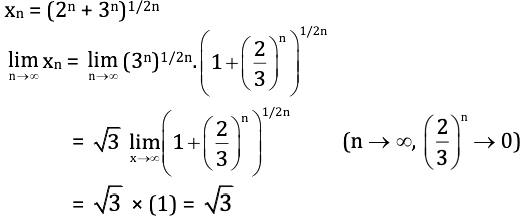Question 14: One of the solutions of equation 8 sin3 θ – 7 sinθ + √3 cos θ = 0 lies in the interval

1. a. (0°, 10°]
2. b. (10°, 20°]
3. c. (20°, 30°]
4. d. (30°, 40°]

Solution:

Given, 8 sin3 θ – 7 sin θ + √3 cos θ = 0

2 . 4 sin3 θ – 7 sin θ + √3 cos θ = 0

2 (3 sinθ – sin 3θ) – 7 sin θ + √3 cos θ = 0

{sin 3θ = 3 sin θ – 4 sin3 θ ⇒ 4 sin3 θ = 3 sin θ – sin 3θ}

√3 cos θ – sin θ – 2 sin 3θ = 0

[√3 / ]2 cos θ – [1 / 2] sin θ = sin 3θ

sin (π / 3) cos θ – cos (π / 3) sin θ = sin 3θ (sin (A – B) = sin A cos B – cos A sin B)

sin [(π / 3) - θ] = 3θ

(π / 3) - θ = nπ + (–1)n

for n = 0

(π / 3) – θ = 3θ

θ = π / 12 = 15° ∈ (10°, 20°]

Question 15: Let a, b, c, d, e, be real numbers such that a + b < c + d, b + c < d + e, c + d < e + a, d + e < a + b. Then

1. a. The largest is a and the smallest is b
2. b. The largest is a and the smallest is c
3. c. The largest is c and the smallest is e
4. d. The largest is c and the smallest is b

Solution:

a + b < c + d …(i)

b + c < d + e …(ii)

c + d < e + a …(iii)

d + e < a + b …(iv)

From (i) & (iii)

a + b < e + a (x < y & y < z ⇒ x < z)

b < e

From (i) & (iv)

d + e < c + d (x < y & y < z ⇒ x < z)

e < c

From (ii) & (iv)

b + c < a + b (x < y & y < z ⇒ x < z)

c < a

b < e < c < a … (1)

Again from (i)

a + b < c + d

(a – c) + b < d

Positive real number + b < d {as a > c}

d > b … (2)

From (iii)

c + d < e + a

(c – e) + d < a

Positive real number + d < a {as c > e}

d < a … (3)

From (1), (2) & (3), we can say that a is largest and b is smallest.

Question 16: If a fair coin is tossed 5 times, the probability that heads does not occur two or more times in a row is:

1. a. 12 / 25
2. b. 13 / 25
3. c. 14 / 25
4. d. 15 / 25

Solution:

The following are the cases for no two heads to come together

Case - I: There is no Head

{TTTTT}

Probability = (1 / 2)5

Case - II: Exactly one Head

{HTTTT, THTTT, TTHTT, TTTHT, TTTTH}

Probability = 5 * (1 / 2)4 (1 / 2) = 5 / 32

Case - III: Exactly Two Heads

{HTHTT, HTTHT, HTTTH, THTTH, THTHT, TTHTH}

Probability = 6 × (1 / 2)3 (1 / 2)2 = 6 / 32

Case - IV: Exactly Three Heads

{HTHTH}

Probability = 1 × (1 / 2)2 * (1 / 2)3 = 1 / 32

Total probability = 13 / 25

Question 17: Consider the following parametric equation of a curve:

x (θ) = | cos 4 θ | cos θ

y (θ) = | cos 4 θ | sin θ

for 0 ≤ θ ≤ 2π

Which one of the following graphs represents the curve?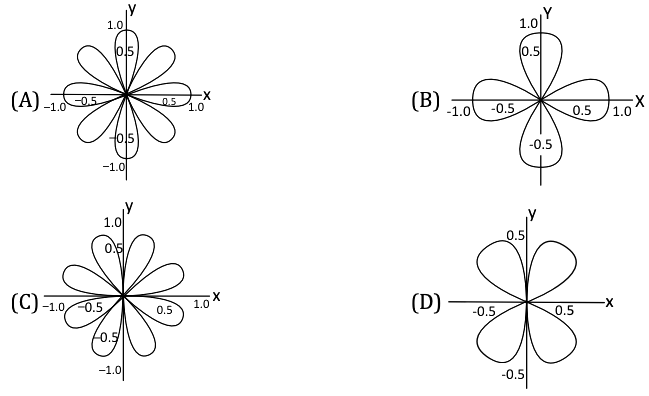Solution:

x (θ) = | cos 4 θ | cos θ

y (θ) = | cos 4 θ | sin θ

where, 0 ≤ θ ≤ 2π

For θ = 0°

x = 1 and y = 0

(1, 0) lies on the curve

Option (c) and (d) are rejected.

For θ = π / 4

x = y = 1 / √2

(1 / √2, 1 / √2) lies on the curve.

Option (b) is rejected.

So, the correct option is (a).

Question 18: Let A = (a1, a2) and B = (b1, b2) be two points in the plane with integer coordinates. Which one of the following is not a possible value of the distance between A and B?

1. a. √65
2. b. √74
3. c. √83
4. d. √97

Solution:

Distance between A (a1, b1) and B (a2, b2)

AB = √(a1 - b1)2 + (a2 - b2)2{Using Distance Formula}

Given a1, a2, b1, b2 ∈ I ⇒ (a1 – b1), (a2 – b2) ∈ I

Now, √65 = √82 + 12 → Possible

√74 = √72 + 52 → Possible

√97 = √92 + 42 → Possible

√83 → Not Possible

Question 19: Let f (x) = max {3, x2, (1 / x2)} for (1 / 2) ≤ x ≤ 2. Then the value of the integral ∫1/22 f(x)dx is:

1. a. 11 / 3
2. b. 13 / 3
3. c. 14 / 3
4. d. 16 / 3

Solution:

f (x) = max {3, x2, (1 / x2)} for (1 / 2) ≤ x ≤ 2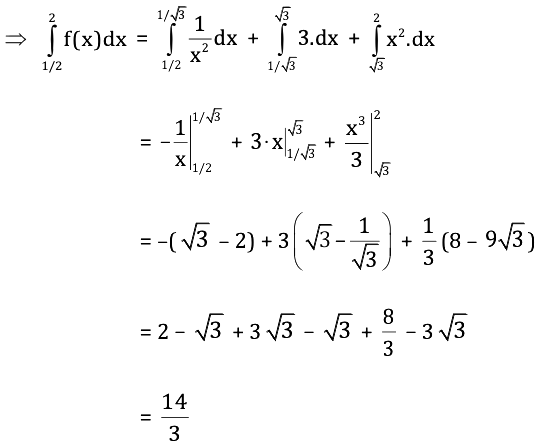Question 20: Let ai = I + (1 / i) for I = 1, 2,………., 20. Put p = (1 / 20) (a1 + a2 + …….. a20) and q = (1 / 20) [(1 / a1) + (1 / a2) + …… (1 / a20). Then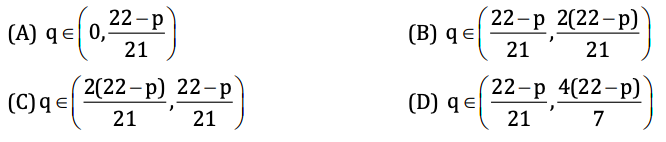Solution:

Clearly, q > 0

We will try to contra prove that q < [22 – p] / 21 OR q + (p / 21) < (22 / 21)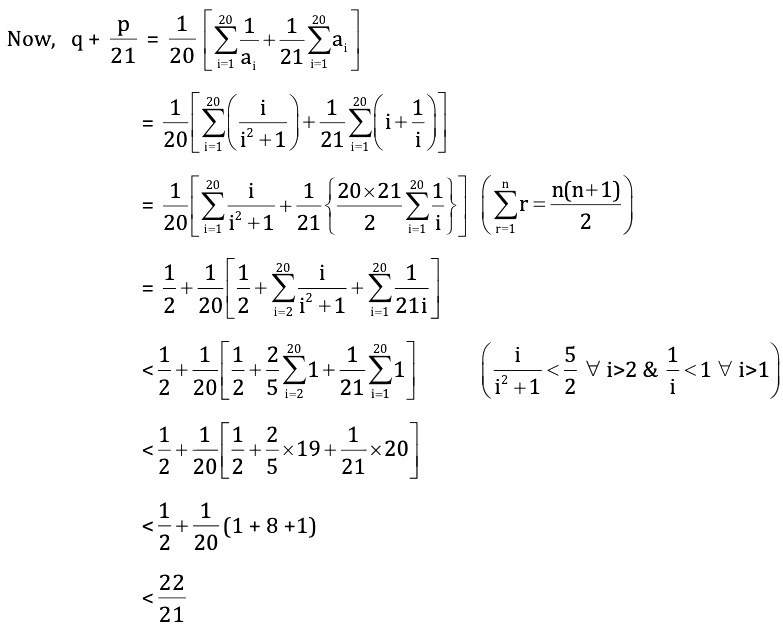Question 21: Let x, y, z be positive integers such that HCF (x, y, z) = 1 and x2 + y2 = 2z2. Which of the following statements are true?

I. 4 divides x or 4 divides y.

II. 3 divides x + y or 3 divides x – y.

III. 5 divides z (x2 – y2)

1. a. I and II only
2. b. II and III only
3. c. II only
4. d. III only

Solution:

Given x, y, z ∈ Z+

HCF (x, y, z) = 1 ⇒ x, y and z are co-prime numbers

x2 + y2 = 2z2 = z2 + z2 ⇒ Sum of the squares of 2 co-prime numbers.

Thus, x = 7, y = 1, z = 5 or x = 1, y = 7, z = 5 are possible combinations.

i.e. 2z2 = 2 . 52 = 50 and x2 + y2 = 72 + 12 = 49 + 1 = 50

Now, x – y is divisible by 3 and z (x2 – y2) is divisible by 5.

Statement II and III are true.

Question 22: How many different (mutually non-congruent) trapeziums can be constructed using four distinct side lengths from the set {1, 3, 4, 5, 6}?

1. a. 5
2. b. 11
3. c. 15
4. d. 30

Solution:

Here, the sides a, b, c, d ∈ {1, 3, 4, 5, 6}

Condition of trapezium,

|a – c| < |b – d| < (a + c)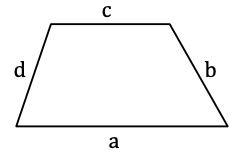Cases:

 (a, c) (b, d) (1, 3) (4, 5), (5, 6) = 2 (1, 4) Not possible (1, 5) Not possible (1, 6) Not possible (3, 4) (1, 5), (1, 6) = 2 (3, 5) (1, 4), (1, 6) = 2 (3, 6) (1, 5) = 1 (4, 5) (1, 3) , (1, 6), (3, 6) = 2 Repeated (4, 6) (1, 5) = 1 (5, 6) (1, 3) , (1, 4) = 1 Repeated

Total number of trapezium = 11

Question 23: A solid hemisphere is mounted on a solid cylinder, both having equal radii. If the whole solid is to have a fixed surface area and the maximum possible volume, then the ratio of the height of the cylinder to the common radius is

1. a. 1 : 1
2. b. 1 : 2
3. c. 2 : 1
4. d. √2 : 1

Solution:

Let Radius of hemisphere and cylinder is R height of cylinder be h.

The whole surface area, S = 2πR2 + 2πRh + πR2

S = 2πRh + 3πR2 …(1)

S – 3πR2 = 2πRh

h = S – 3πR2 / 2πR …(2)

Now, V = (2 / 3) πR3 + πR2h

V = (2 / 3) πR3 + πR2 [S – 3πR2 / 2πR] {from eq.(2)}

Differentiate with respect to R

dV / dR = 2πR2 + (S / 2) - (9 / 2) πR2

For maximum and minimum dV / dR = 0

0 = 2πR2 + (S / 2) - (9 / 2) πR2

0 = –5πR2 + S

5πR2 = S

5πR2 = 3πR2 + 2πRh {from eq.(1)}

5R = 3R + 2h

2R = 2h

h : R = 1 : 1

Question 24: Let ABC be an acute scalene triangle, and O and H be its circumcentre and orthocenter respectively. Further, let N be the midpoint of OH. The value of the vector sum NA + NB + NC is: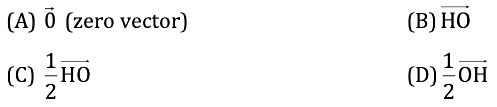Solution:

Let circumcenter O of triangle ABC be at the origin.

Position vector of Centroid, G = [a + b + c] / 3

Now, we know that centroid(G) divides the distance from the orthocenter (H) to the circumcenter(O) in the ratio 2:1.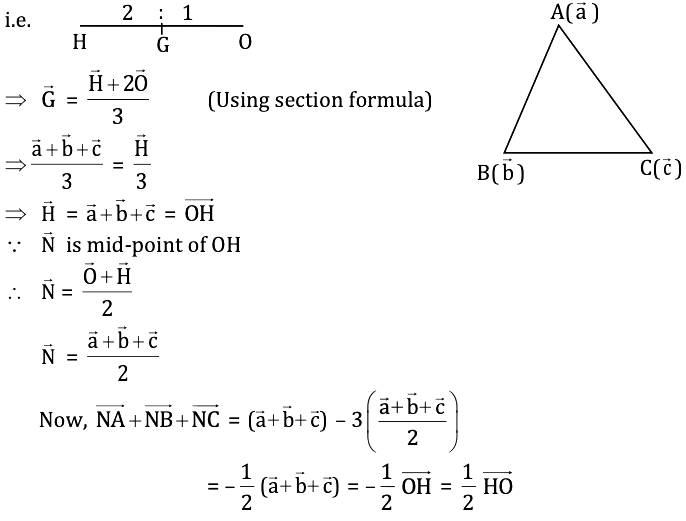Question 25: The quotient when 1 + x2 + x4 + x6 + ………..x34 is divided by 1 + x + x2 + x3 +......+ x17 is

1. a. x17 – x15 + x13 – x11...... + x
2. b. x17 + x15 + x13 + x11...... + x
3. c. x17 + x16 + x15 + x14...... + x
4. d. x17 – x16 + x15 – x14...... – x

Solution:

1 + x2 + x4 + x6 + ………..x34 (Forms a G.P with common ratio x2)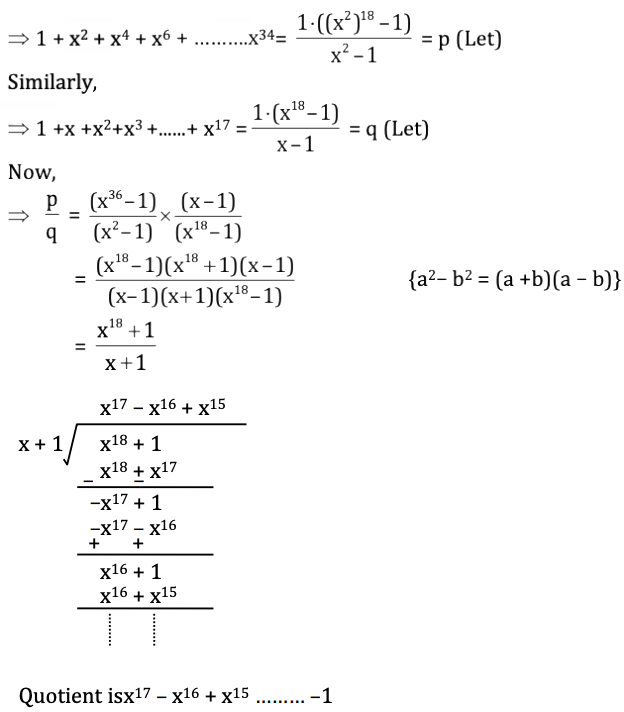Question 26: Let R be the region of the disc x2 + y2 ≤ 1 in the first quadrant. Then the area of the largest possible circle contained in R is

1. a. π (3 − 2√2)
2. b. π (4 − 3√2)
3. c. π / 6
4. d. π (2√2 - 2)

Solution:

Let the radius of inner circle = r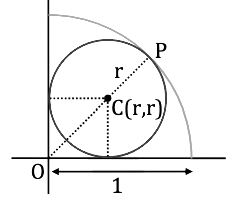This inner circle touches both the axes,

Centre of circle = (r, r)

Now, OP = OC + CP

1 = √(r2 + r2) + r {Using Distance Formula}

1 = √2r + r

r = 1 / √(2) + 1

r = [1 / √(2) + 1] * [√(2) - 1 / √(2) - 1]

r = √(2) - 1 / 1 = √(2) - 1

Area of Circle = πr2

= π (√(2) - 1)2

= π (2 + 1 - 2√2)

= π (3 - 2√2)

Question 27: Let R be the set of real numbers and f : R → R be given by f (x) = √|x| - log (1 + |x|). We now make the following assertions:

I. There exists a real number A such that f (x) ≤ A for all x.

II. There exists a real number B such that f (x) ≥ B for all x.

1. a. I is true and II is false
2. b. I is false and II is true
3. c. I and II both are true
4. d. I and II both are false

Solution:

f (x) = √|x| - log (1 + |x|)

For x > 0

f (x) = x – log (1 + x)

On differentiating both sides with respect to x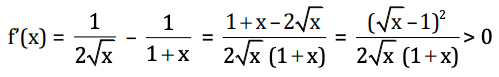f (x) is increasing when x > 0 …(i)

Also, f (0) = √|0| – log (1 + |0|) = 0 ...(ii) &

f (–x) = √|–x| – log (1 + |–x|) = f (x)

f(x) is Even Function ...(iii)

Therefore, using (i), (ii) and (iii) graph of f (x) can be drawn as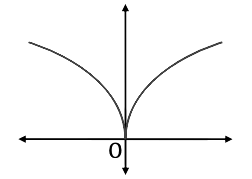Clearly, f (x) is continuous everywhere but non-differentiable at x = 0.

Question 28: Define g(x) = ∫-33 f (x - y) f (y) dy, for all real x, where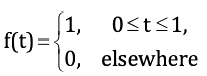Then

1. a. g (x) is not continuous everywhere
2. b. g (x) is continuous everywhere but differentiable nowhere
3. c. g (x) is continuous everywhere and differentiable everywhere except at x = 0, 1
4. d. g (x) is continuous everywhere and differentiable everywhere except at x = 0, 1, 2

Solution:

Given, ∫-33 f (x - y) f (y) dy and f (y) =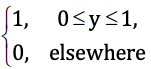g (x) = ∫01 f (x – y) dy

Now, put x – y = t

dy = –dt

Lower limit, y = 0 ; t = x

Upper limit, y = 1 ; t = x – 1

g (x) = – ∫xx-1 f (t) dt {Using property: – ∫ab f (x) dx = ∫ba f (x) dx}

g (x) = ∫x-1x f (t) dt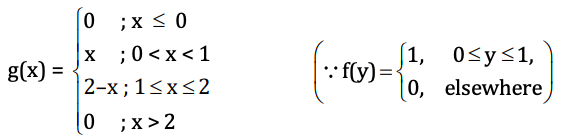g (x) is continuous everywhere, but non-diff. at x = 0, 1, 2 (see figure)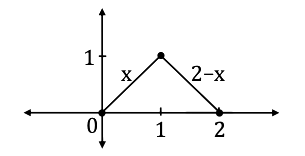Question 29: The integer part of the number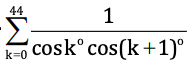is

1. a. 50
2. b. 52
3. c. 57
4. d. 59

Solution: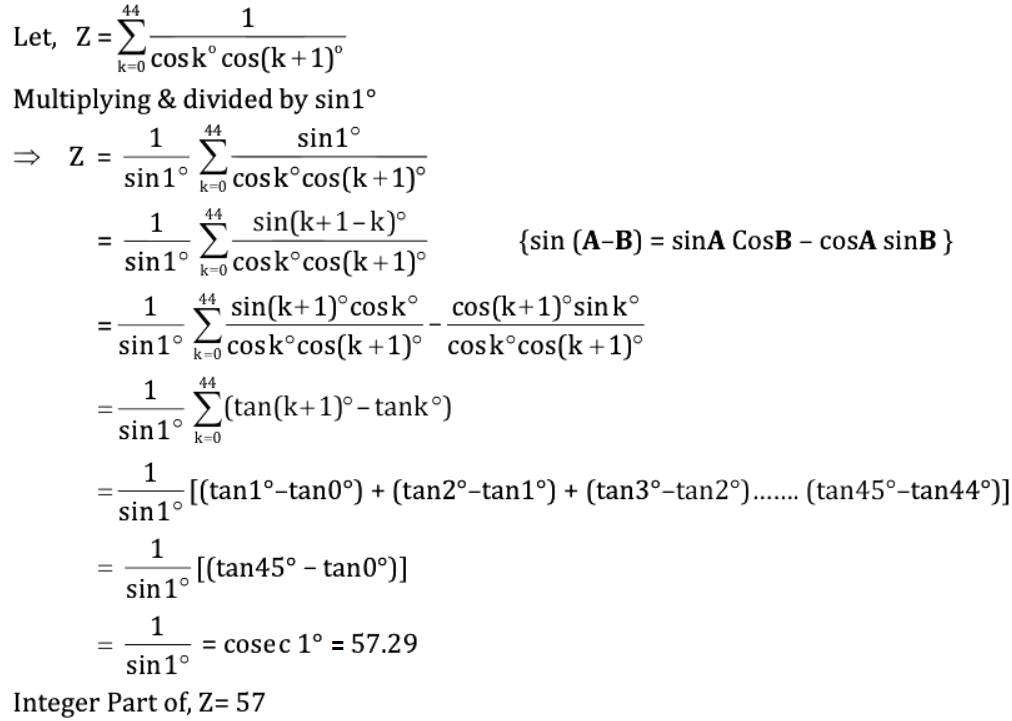Question 30: The number of continuous functions f : [0, 1] → R that satisfy

1. a. 0
2. b. 1
3. c. 2
4. d. infinity

Solution: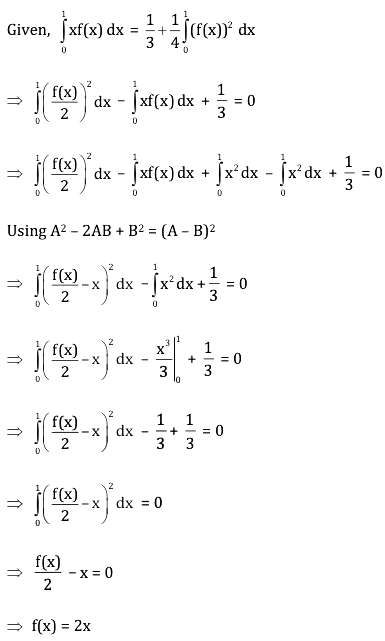Therefore, the number of such continuous functions is 1.

### KVPY SX 2017 Maths Paper with Solutions